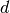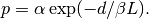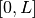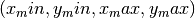Warning

This documents an unmaintained version of NetworkX. Please upgrade to a maintained version and see the current NetworkX documentation.

# waxman_graph¶

waxman_graph(n, alpha=0.4, beta=0.1, L=None, domain=(0, 0, 1, 1))[source]

Return a Waxman random graph.

The Waxman random graph model places n nodes uniformly at random in a rectangular domain. Each pair of nodes at Euclidean distanceis joined by an edge with probabilityThis function implements both Waxman models, using the L keyword argument.

• Waxman-1: if L is not specified, it is set to be the maximum distance between any pair of nodes.
• Waxman-2: if L is specified, the distance between a pair of nodes is chosen uniformly at random from the interval.
Parameters: n (int) – Number of nodes alpha (float) – Model parameter beta (float) – Model parameter L (float, optional) – Maximum distance between nodes. If not specified, the actual distance is calculated. domain (four-tuple of numbers, optional) – Domain size, given as a tuple of the form. G Graph

References

  B. M. Waxman, Routing of multipoint connections. IEEE J. Select. Areas Commun. 6(9),(1988) 1617-1622.